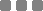# Consolidation Test

รหัสสินค้า : Consolidation Test

USAGE OF THE CONSOLIDATION MACHINE

This machine use for soil press test. It can test soil press and soil transformation, the relation of void ratio with press,

the relation of time with transformation.Then you can calculation the unit of setting volume, compression index, swelling index,

modulus of compression and coefficient of consolidation.

THE MAIN SPECIFICATIONS

For lower press:

The testing sample area is 30cm square, press range is 12.5Kpa to 800Kpa

The testing sample area is 50cm square, press range is 12.5Kpa to 400Kpa

For middle press:

The testing sample area is 30cm square, press range is 12.5Kpa to 1600Kpa

The testing sample area is 50cm square, press range is 12.5Kpa to 800Kpa

For the high press:

The testing sample area is 30cm square, press range is 12.5Kpa-4000Kpa

The testing sample area is 50cm square, press range is 12.5Kpa-2000Kpa

 จำนวน ราคา 0.00 บาท ราคารวม 0.00 บาท

สินค้าไม่เพียงพอ

สินค้าหมด

Visitors: 106,011